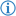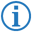The multiply operator is an arithmetic operator which multiplies two number values together.

Its token is named MULTIPLICATION.

## Phrases

### InfixInfix notation can be achieved using the phrases `multiplied with` or `times`.

Its FDS is

#### Example

`I wrote`` ``the number`` ``6`` ``multiplied by`` ``the number`` ``8``.`

This prints `48`.

Its Java equivalent is as follows:

```System.out.println((double)6 * (double)8);
```

### PrefixPrefix notation can be achieved using the phrase `multiply` or `the product of` and the infix separator `by` or `and`.

Its FDS is

#### Example

`I wrote`` ``multiply`` ``the number`` ``6`` ``by`` ``the number`` ``8``.`

This has the same effect as the above example.